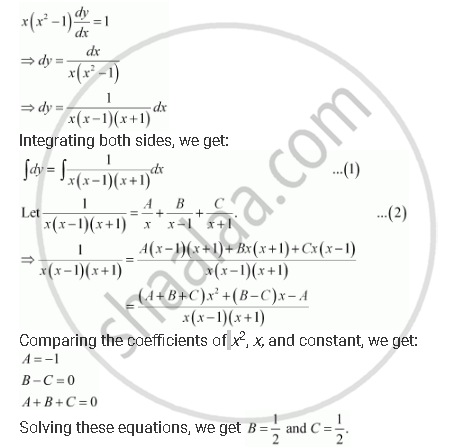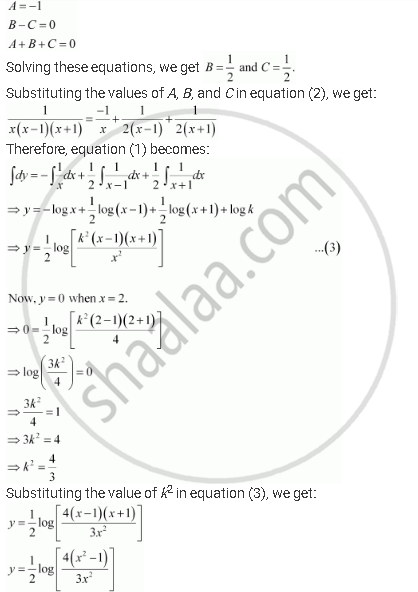Share

# For Each of the Differential Equations Find a Particular Solution Satisfying the Given Condition: X(X^2 - 1) Dy/Dx = 1 , Y = 0 " When X " = 2 - CBSE (Commerce) Class 12 - Mathematics

ConceptMethods of Solving First Order, First Degree Differential Equations Differential Equations with Variables Separable

#### Question

For each of the differential equations find a particular solution satisfying the given condition:

x(x^2 - 1) dy/dx = 1 , y = 0 " when x " = 2

#### SolutionComparing the coefficients of x2x, and constant, we get:Is there an error in this question or solution?

#### APPEARS IN

NCERT Solution for Mathematics Textbook for Class 12 (2018 to Current)
Chapter 9: Differential Equations
Q: 12 | Page no. 396
Solution For Each of the Differential Equations Find a Particular Solution Satisfying the Given Condition: X(X^2 - 1) Dy/Dx = 1 , Y = 0 " When X " = 2 Concept: Methods of Solving First Order, First Degree Differential Equations - Differential Equations with Variables Separable.
S